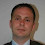## Wednesday, March 2, 2011

### Is it safe to move lower bounds to zero?

Assume we have the problem

min c'x
st.   A x = b        (P0)
x >= l

where l is large in absolute value say l_j=-1000 for all js. (l is short for lower bounds). The dual problem is

max b'y + l's
st.    A'y + s = c  (D0)
s >= 0

It is very common to transform the problem to

min c'x + c'l
st.   A x = b- A l  (P1)
x >= 0

for efficiency reasons. Indeed all interior-point optimizers will do that.

The dual problem is

max b'y + c'l
st.    A'y + s = c  (D1)
s >= 0

Let us say we solve (P1) and (D1). Moreover,

A'y + s =  c

holds only approximate which definitely be the case for interior-point methods. To be precise we have

A'y + s =  c + e

holds exactly where 0 < |e|| << 1.

This implies if (y,s) from (D1) is reported as an optimal solution to (D0) then there can be a big error in the dual objective value. Note that is not the case if l=0. Now if instead we report (y,c-A'y) as the optimal dual solution to (D0) then objective value will be correct but then s>=0 might be violated.

The question is that which dual solution to report. I guess the answer is that it depends on your priorities.

I will leave it as an exercise to the interested reader to construct a small example demonstrating this. Since I just spend all day figuring out that happening on instance with 100K variables.

#### 1 comment:

1.Interesting. I have had similar experiences, to some extent it's a bad formulation and will only happen with large bounds. In my opinion returning a dual solution which does not validate feasibility is preferred. If the primal and dual objectives differs significantly, then the user must deal with it him self. It's a no win situation for the optimizer vendor..